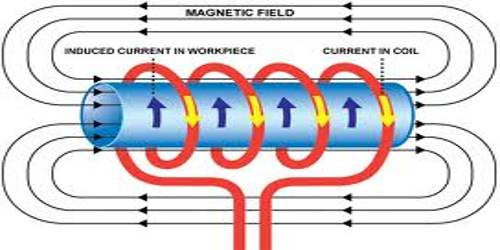# Laws of Electromagnetic Induction

Laws of electromagnetic induction:

Electro-magnetic induction is the production of an electromotive force across an electrical conductor in a changing magnetic field. So, the process of creating electromotive force and current in a closed coil of the wire due to the influence of a moving magnet or current-carrying coil is called electromagnetic induction.

Scientist Michael Faraday postulated laws of electromagnetic inductions According to his name the laws are known Faraday’s Laws of electromagnetic induction. These laws are stated below:

First law: Whenever there is a change of a number of magnetic lines of induction or magnetic flux in a closed loop, then the electromotive force is induced in the loop. Whenever the magnetic flux linked with a circuit changes, an e.m.f. is always induced in it. This means that the current induced by a decrease in magnetic flux produces a magnetic field that increases the magnetic flux.

Second law: The value of the induced electromotive force in a soil is directly proportional to the rate of change at a number of magnetic field lines or magnetic flux. The magnitude of the induced e.m.f. is equal to the rate of change of flux linkages. This means that the current induced by a decrease in magnetic flux produces a magnetic field that increases the magnetic flux.